Consider two waves with equal frequency and amplitude,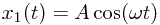and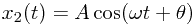.

The difference between these two waves can be computed as follows: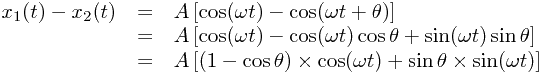Now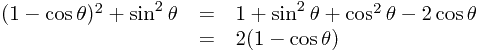so we may write: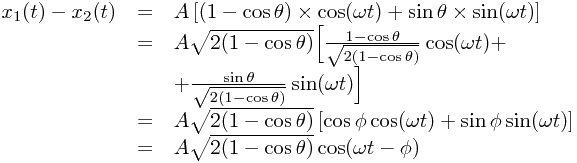where we have calculated a new angleusing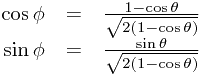These equations are consistent as a consequence of the division by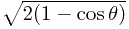.

Hence the maximum value of the difference is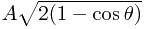, which is achieved at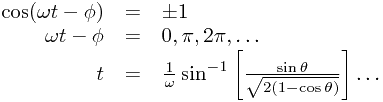Thus given the maximum distance apart of two out-of-phase but otherwise identical oscillators, we simply say: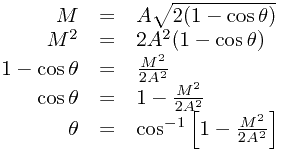Finally, in the case of the maximum being half the amplitude,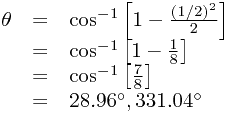# Phase Differences

## Discrepancies in sinusoidal waves

© Carl Turner 2008-2017